# Reelin' n Boppin'

## Slot Machine published 12 years ago by Aristocrat Leisure Industries Pty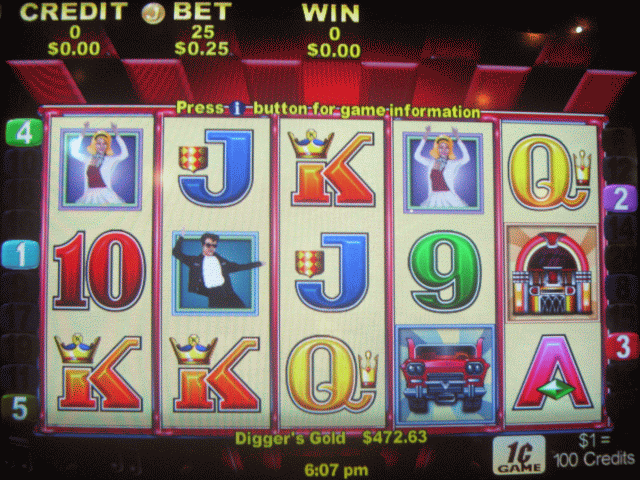## Not listed in MAME yet.
Info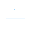(0)
Edit
Upload
### Reelin' n Boppin' © 2008 Aristocrat.

A 5-reel video slot machine with a 1950's theme.

### TECHNICAL INFORMATION

1
Aristocrat MKVI hardware.

Video resolution : 640x480

### TRIVIA

This game has updated graphics over its predecessor "Reelin-n-Rockin [20 Lines]". A jukebox replaces the gumball machine on the original game. Every symbol was remade, right down to the record animation. The sound effects remained unaltered.

### SCORING

Car : 3 = 500, 4 = 1000, 5 = 2500
Jukebox : 3 = 100, 4 = 500, 5 = 2000
Girl : 3 = 100, 4 = 200, 5 = 1000
Man : 3 = 50, 4 = 100, 5 = 1000
Ace : 3 = 20, 4 = 100, 5 = 200
King : 3 = 15, 4 = 50, 5 = 200
Queen : 3 = 10, 4 = 20, 5 = 100
Jack : 3 = 10, 4 = 20, 5 = 100
Ten : 2 = 5, 3 = 10, 4 = 20, 5 = 100
Nine : 2 = 5, 3 = 10, 4 = 20, 5 = 100
Record : 2 = 2, 3 = 5, 4 = 20, 5 = 50

### TIPS AND TRICKS

* All pays are left to right, except scatters which pay any.

* Grill appears on reels 2 and 4 and substitutes for all symbols.

* Feature : Spin up 3, 4 or 5 Records to trigger 20, 30 or 40 free games respectively.

* During the free games the Grill substitutes are x2 and/or x3 respectively. If both symbols substitute in a win it is x6.

### SERIES

1) Reelin-n-Rockin (1998)
2) Indian Dreaming (1999)
3) Reelin-n-Rockin [Multi-line] (2000)
4) Indian Dreaming [Multi-line] (2000)
5) Pompeii (2001)
6) Mystic Power (2002)
7) Thai Princess (2002)
8) Dream Catcher (2002)
9) Indian Dreaming [Lady Luck] (2003)
10) Indian Dreaming 2nd Chance (2003)
11) Pride of Africa (2005)
12) Wild Tepee [Power Pay] (2007)
13) Reelin' n Boppin' (2008)